Mobile QR Code1. (Chungbuk National University, Korea)

Coarse-fine, Digital LDO, oscillation, settling time, voltage differential detector.

## I. INTRODUCTION

Portable devices, biomedical devices, and IoT applications required the low power consumption in accordance with the small batteries. Fine-grain point-of-load power management is used in digital integrated circuits to enhance the power efficiency by using multiple supply-voltages. A digital low-dropout regulator (D-LDO) is suitable for a device with low power consumption -.

Fig. 1 shows the conventional D-LDO consisting of a comparator, a bidirectional shift-register, and a PMOS array. It is implemented with digital circuits to improve power efficiency by removing the static power consumption. At first, the comparator detects the difference between the output voltage ($V_{\mathrm{OUT}}$) and the reference voltage ($V_{\mathrm{REF}}$). Then, according to the comparator output result, the bidirectional shift-register turns on or off a PMOS transistor per a clock cycle, When the D-LDO regulates $V_{\mathrm{OUT}}$ to $V_{\mathrm{REF}}$, a PMOS transistor turns on and off repeatedly per a clock cycle.

Fig. 1. (a) Schematic and (b) waveforms of the conventional digital low-dropout regulator (D-LDO).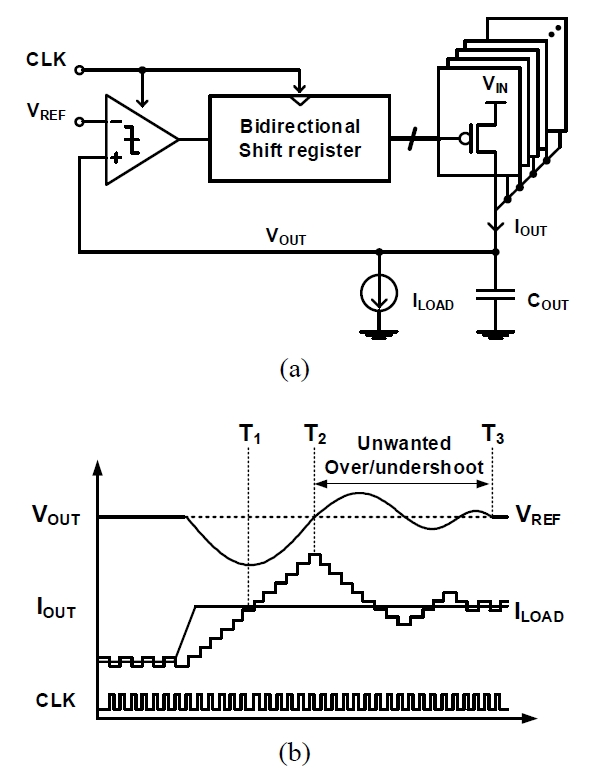When the light-to-heavy load current ($I_{\mathrm{LOAD}}$) transition occurs as shown in Fig. 1(b), a large undershoot voltage is formed. The D-LDO turns on PMOS transistors to recover $V_{\mathrm{OUT}}$ to $V_{\mathrm{REF}}$. At time $T\_{1}$, the output current ($I_{\mathrm{OUT}}$) is equal to $I_{\mathrm{LOAD}}$, but $V_{\mathrm{OUT}}$ falls to its minimum voltage. Thus, $I_{\mathrm{OUT}}$ increases until $V_{\mathrm{OUT}}$ exceeds $V_{\mathrm{REF}}$. At time $T_{2}$, $I_{\mathrm{OUT}}$ is about two times $I_{\mathrm{LOAD}}$. This increases $V_{\mathrm{OUT}}$, which causes an unwanted overshoot voltage. The unwanted overshoot voltage also generates an unwanted undershoot voltage. In this way, the unwanted overshoot and undershoot voltages occur until $V_{\mathrm{OUT}}$ settles to $V_{\mathrm{REF}}$ at time $T_{3}$. This leads to a long settling time.

The coarse-fine D-LDO  reduced both the settling time and the quiescent current by using coarse and fine control loops, respectively. When a large load current transition occurs, it turns on or off a large PMOS transistor per a fast clock cycle in the coarse mode to reduce the settling time. However, this causes a transient oscillation (unwanted overshoot and undershoot voltages), which prevents the D-LDO from triggering the fine mode. In order to re-activate the fine mode, the coarse-fine DLDO must insert a guard time (the maximum settling time) in the coarse control loop. Therefore, the settling time instead increases.

In this paper, the proposed D-LDO removes the transient oscillation by using a voltage differential detector and by dividing the coarse mode into matching and tracking modes. Section II describes the architecture of the proposed coarse-fine D-LDO. Section III shows the simulation results. This paper draws conclusions in Section IV.

## II. ARCHITECTURE

Fig. 2 shows the proposed coarse-fine D-LDO with a voltage differential detector. It reduces the settling time and quiescent current by using the coarse and fine power stages, respectively. These two power stages adjust the output current ($I_{\mathrm{OUT}}$) to the load current ($I_{\mathrm{LOAD}}$) coarsely and finely using the coarse and fine PMOS array currents ($I_{\mathrm{COARSE}}$ and $I_{\mathrm{FINE}}$), respectively, which consist of the coarse and fine PMOS arrays driven by the coarse and fine bidirectional shift-registers (Bi-SRs), respectively. Each power stage is controlled by a comparator (CMP1), a digital controller, a coarse mode detector, and a voltage differential detector. As shown in Fig. 3, the D-LDO uses the comparator with a comparison done signal (DONE) in order to reduce the delay between the Bi-SRs and the comparators by operating the Bi-SRs as soon as the comparison is done . The unit PMOS transistor in the coarse PMOS array is equal to N times of the unit PMOS transistor in the fine PMOS array.

Fig. 4 shows the operation waveforms of the proposed D-LDO. In steady state, the D-LDO finely adjusts $I_{\mathrm{OUT}}$ using the small PMOS transistors and the slow clock frequency at the fine mode. The CMP1 compares the output voltage ($V_{\mathrm{OUT}}$) with the reference voltage ($V_{\mathrm{REF}}$) at the comparator clock frequency (CLK_CMP). After the comparison, the fine power stage turns on or off a small unit PMOS transistor of the fine PMOS array per a clock cycle, according to the comparator output signal (CMP_F) and the comparison done signal (CLK_Fine).

When $I_{\mathrm{LOAD}}$ changes largely, the coarse mode detector senses the overshoot or undershoot exceeding the boundary voltages ($V_{\mathrm{REF\_H}}$ and $V_{\mathrm{REF\_L}}$) at a fast clock frequency (CLK_Fast). The D-LDO then coarsely adjusts $I_{\mathrm{OUT}}$ with CLK_Fast at the coarse mode. The voltage differential detector is activated by the coarse mode detector with a voltage differential detector clock frequency (CLK_VD) during the coarse mode.

The coarse power stage turns on or off a large unit PMOS transistor of the coarse PMOS array per a clock cycle according to the increase signal (INC) and the voltage differential signal (VD). The coarse mode is divided into matching and tracking modes. The matching mode rapidly matches the output current to the load current with the fast clock frequency by using the voltage differential detector to reduce the overshoot and undershoot voltages. Meanwhile, the tracking mode tracks the output voltage to the reference voltage with the slow clock frequency to remove unwanted overshoot and undershoot voltages.

When $I_{\mathrm{LOAD}}$ changes from light to heavy, $V_{\mathrm{OUT}}$ decreases below $V_{\mathrm{REF\_L}}$. In this case, INC becomes high and VD becomes low. The D-LDO operates in the matching mode. It turns on a large unit PMOS transistor per a clock cycle at the fast clock frequency in order to reduce the undershoot voltage. When $I_{\mathrm{OUT}}$ is over $I_{\mathrm{LOAD}}$, VD becomes high. At this time, $I_{\mathrm{OUT}}$ is equal to $I_{\mathrm{LOAD}}$ but $V_{\mathrm{OUT}}$ falls to its minimum voltage. Without the tracking mode, $I_{\mathrm{OUT}}$ settles to $I_{\mathrm{LOAD}}$ and $V_{\mathrm{OUT}}$ becomes a nearby minimum voltage. However, the D-LDO operates in the tracking mode. It coarsely increases $I_{\mathrm{OUT}}$ at the slow frequency until $V_{\mathrm{OUT}}$ is settled within $V_{\mathrm{REF\_H}}$ and $V_{\mathrm{REF\_L}}$. Then, the fine mode is retriggered to finely regulate $V_{\mathrm{OUT}}$ to $V_{\mathrm{REF}}$. When $I_{\mathrm{LOAD}}$ changes from heavy to light, the D-LDO performs the opposite operation of the undershoot voltage generation. As a result, the proposed D-LDO not only reduces the undershoot and overshoot voltages, but also removes the transient oscillation shown in Fig. 1.

Fig. 5. Operation waveforms of the proposed voltage differential detector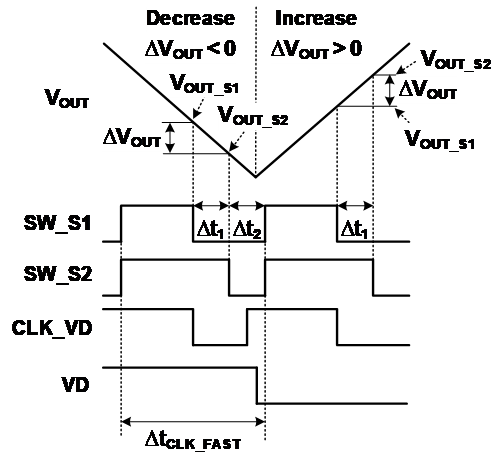Fig. 5 shows the waveforms of the proposed voltage differential detector in Fig. 2. At first, the two sampling signals (SW\_S1 and SW_S2) sequentially sample two output voltages ($V_{\mathrm{OUT\_S1}}$ and $V_{\mathrm{OUT\_S2}}$) into the capacitors ($C_{1}$ and $C_{2}$). The CMP2 then compares $V_{\mathrm{OUT\_S1}}$ with $V_{\mathrm{OUT\_S2}}$ at the rising edge of CLK_VD. VD becomes either low or high based on whether $V_{\mathrm{OUT}}$ decreases (${\Delta}$$V_{\mathrm{OUT}} < 0) or increases ({\Delta}$$V_{\mathrm{OUT}}$ > 0) during the time interval (${\Delta}$t$_{1}$), respectively.

The clock frequency of SW_S1 is set to be equal to the clock frequency of CLK_FAST to improve the transient response. The time intervals ${\Delta}$t$_{1}$ and ${\Delta}$t$_{2}$ are designed to be a quarter of the period of CLK_FAST to ensure tracking $V_{\mathrm{OUT\_S2 }}$to $V_{\mathrm{OUT}}$ and comparing $V_{\mathrm{OUT\_S1}}$ with $V_{\mathrm{OUT\_S2}}$, respectively.

Fig. 6. Operation waveforms of the comparator with the comparison done signal.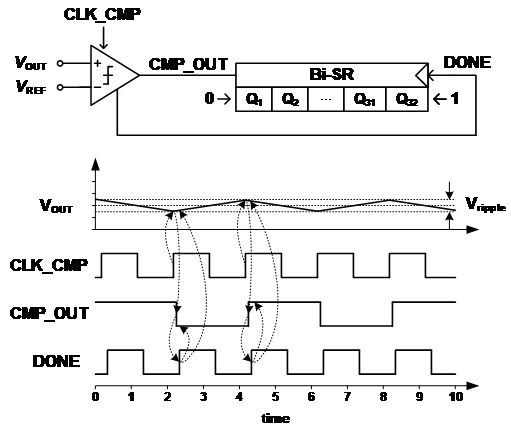Fig. 6 shows the operation of the comparator with the completion done signal (DONE) . When the comparator compares VOUT with VREF, DONE becomes high as soon as the comparison complete. The bidirectional shift-register (Bi-SR) then shifts the data to right or left according to CMP_OUT by DONE signal. This decreases the output ripple voltage ($V_{\mathrm{ripple}}$).

## III. SIMULATION RESULT

The proposed D-LDO is designed with a 65nm CMOS process. Fig. 7 shows the layout of the proposed D-LDO, which has an area of 3,074${\mathrm{\mu}}$m$^{2}$. Fig. 8 shows the simulated steady-state output ripple voltage when the load current changes from 2mA to 12mA at $V_{\mathrm{IN}}$=500mV. The proposed D-LDO regulates $V_{\mathrm{OUT}}$ to 450mV from $V_{\mathrm{IN}}$ of 500mV. It has a quiescent current of 7.04μA, including the dynamic current of the comparator, which is of 0.46μA. $V_{\mathrm{REF\_H}}$ and $V_{\mathrm{REF\_L}}$ are 15mV above and below $V_{\mathrm{REF}}$ of 450mV, respectively. The output capacitor is 1nF. CLK_Slow is 20MHz and CLK_Fast is 100MHz. The proposed D-LDO has output ripple voltages of 0.6mV-3.7mV. It reduces the output ripple voltages by using the comparator with the comparison done signal.

Fig. 8. Simulated steady-state output ripple voltage, when the load current changes from 2mA to 12mA at $V_{IN}$=500mV.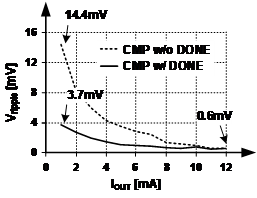Fig. 9. Simulated waveforms of (a) conventional D-LDO  and (b) proposed D-LDO.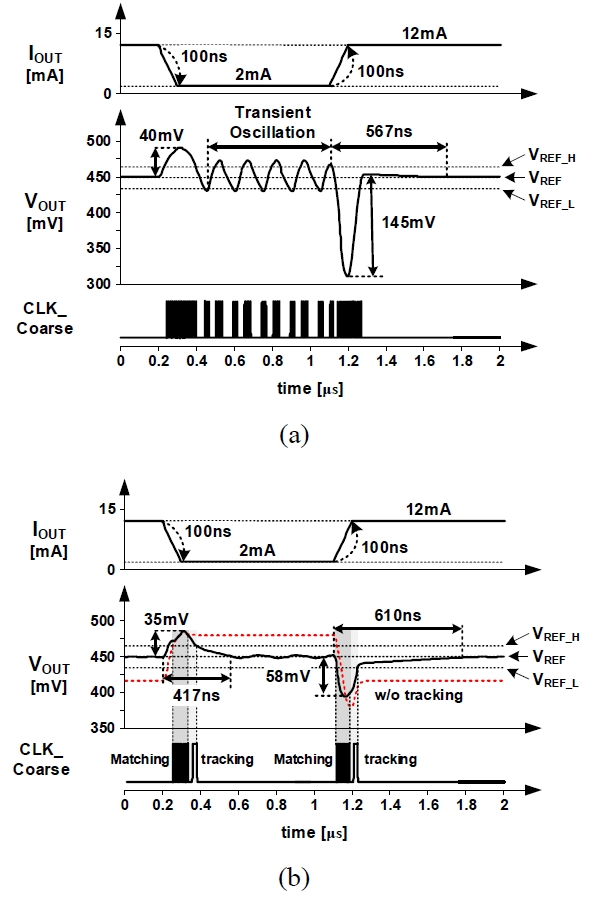Table 1. Comparison results of D-LDOs

 Conventional D-LDO  Proposed D-LDO Process 65nm CMOS Input voltage 500mV Output voltage 450mV Clock frequency 20MHz&100MHz $C_{OUT}$ 1nF Load step 2-12mA Edge time 100ns Load regulation 0.03mV/mA Line regulation 0.2mV/V Quiescent current 6.61μA 7.04μA Peak current eff. 99.9% 99.9% Vripple 0.6-14.4mV 0.6-3.7mV Overshoot 40mV 35mV Undershoot 145mV 58mV Settling time (1% accuracy) Oscillation @overshoot 567ns @undershoot 417ns @overshoot 610ns @undershoot

Fig. 9 and Table 1 show the simulated waveforms and comparison results of the conventional  and proposed D-LDOs. When $I_{\mathrm{LOAD}}$ changes from 12mA to 2mA within the edge time of 100ns, the conventional D-LDO has the overshoot of 40mV, the undershoot of 145mV, and the settling time of 567ns. However, it causes the transient oscillation at the heavy-to-light load transition. But, the proposed D-LDO removes the transient oscillation by dividing the coarse mode into the matching and tracking modes with the voltage differential detector. It rapidly matches the output current to the load current during the matching mode with the fast clock frequency. Then, it tracks the output voltage to the reference voltage during the tracking mode with the slow clock frequency. Without the tracking mode, $V_{\mathrm{OUT}}$ would settle to almost the maximum and the minimum voltages when the overshoot and undershoot voltages are generated, respectively, due to the channel length modulation effect.

Table 2 shows the performance comparisons of the D-LDOs. The figure-of-merit used for D-LDO  is as follows:

##### (1)
\begin{equation*} FoM_{3}=\frac{I_{Q}}{\Delta I_{LOAD}}\cdot \frac{\Delta V_{OUT}}{V_{OUT}}\cdot \textit{Settling time} \end{equation*}

where $C_{\mathrm{OUT}}$ is the output capacitor, ${\Delta}$$V_{\mathrm{OUT}} is the maximum undershoot voltage, I_{\mathrm{Q}} is the quiescent current, and {\Delta}$$I_{\mathrm{LOAD}}$ is the load current change range. The proposed D-LDO has the smallest FoM.

Table 2. Performance comparison of D-LDOs

 ISSCC 2017  VLSI 2017  JSSC 2017  PE 2018  TCAS Ⅱ 2016  This Work Technology 65nm 130nm 28nm 130nm 65nm 65nm Active area 0.0023$mm^2$ 0.0631$mm^2$ 0.021$mm^2$ 0.014$mm^2$ 0.01$mm^2$ 0.003$mm^2$ Input voltage 0.5-1V 0.84-1.24V 1.1V 0.7-1.2V 0.6-1.1V 0.5V Output voltage ($V_{OUT}$) 0.3-0.45V 0.6-1V 0.9V 0.6-1.1V 0.4-1V 0.45V Max. Load current ($I_{LOAD}$) 2mA 50mA 200mA 25mA 100mA 12mA Quiescent current (IQ) 14μA 400μA 110μA 6μA 82μA 7.04μA Peak current efficiency 99.8% 99.2% 99.94% 99.9% 99.92% 99.93% Load regulation 5.6mV/mA 0.4mV/mA N/A 0.04mV/mA 0.06mV/mA 0.03mV/mA Line regulation 2.3mV/V N/A N/A N/A 3mV/V 0.2mV/V $C_{OUT}$ 0.4nF 0.5nF 23.5nF 1nF 1nF 1nF Edge time < 1ns 10ns 4μs N/A 20ns 100ns $∆V_{OUT}$@$∆I_{LOAD}$ 40mV @1.06mA 250mV @50mA 120mV @180mA 200mV @23.5mA 55mV @98mA 58mV @10mA Settling time 0.1μs 0.25μs >10μs 2.08μs 0.7μs*1 0.61μs FoM*2 117ps 500ps 1600ps 170ps 64ps 55ps

*1 Maximum possible settling time${\approx}$1.2${μ}$s

*2 FoM = ($I_{\mathrm{Q}}$/${\Delta}$$I_{\mathrm{LOAD}}){\cdot}({\Delta}$$V_{\mathrm{OUT}}$/$V_{\mathrm{OUT}}$)${\cdot}$Settling time

## V. CONCLUSIONS

In this paper, the D-LDO with the voltage differential detector for removing the transient oscillation is proposed. It uses the coarse-fine method to reduce both the settling time and the quiescent current. When a large load current transition occurs, the proposed D-LDO matches the output current to the load current during the matching mode. Then, it tracks the output voltage to the reference voltage during the tracking modem, which removes the transient oscillation. It was implemented using a 65nm CMOS process. When the load current transition is 10mA, the overshoot and undershoot voltages are 35mV and 58mV within the settling times of 417ns and 610ns, respectively. The quiescent current is 7.04μA and the peak current efficiency is 99.9%.

### ACKNOWLEDGMENTS

This work was supported by the Ministry of Education, Science and Technology through Basic Science Research Program, National Research Foundation of Korea under Grant 2018R1D1A3B07047986. The chip fabrication and EDA tool were supported by the IC Design Education Center (IDEC), Korea.

### REFERENCES

1
Hazucha P., et al. , April 2005, Area-efficient linear regulator with ultra-fast load regulation, in IEEE Journal of Solid-State Circuits, Vol. 40, No. 4, pp. 933-9402
Lam Y., Ki W., Feb 2008, A 0.9V 0.35 μm Adaptively Biased CMOS LDO Regulator with Fast Transient Response, 2008 IEEE International Solid-State Circuits Conference - Digest of Technical Papers, San Francisco, pp. 442-6263
Okuma Yasuyuki, al. et, Sep 2010, 0.5-V input digital LDO with 98.7% current efficiency and 2.7-µA quiescent current in 65nm CMOS, IEEE Custom Integrated Circuits Conference 2010, pp. 1-44
Huang M., et al. , July 2016, A Fully Integrated Digital LDO With Coarse–Fine-Tuning and Burst-Mode Operation, in IEEE Transactions on Circuits and Systems II: Express Briefs, Vol. 63, No. 7, pp. 683-6875
Woo K., Kim T., Hwang S., Kim M., Yang B., Jan 2018, A fast-transient digital LDO using a double edge-triggered comparator with a completion signal, 2018 International Conference on Electronics, Information, and Communication (ICEIC), pp. 1-46
Salem L. G., Warchall J., Mercier P. P., Feb. 2017, A 100nA-to-2mA successive-approximation digital LDO with PD compensation and sub-LSB duty control achieving a 15.1ns response time at 0.5V, in IEEE Int. Solid-State Circuits Conf. (ISSCC) Dig. Tech. Papers, pp. 5-97
Lim et al. C. Y., Aug 2017, A 50-mA 99.2% Peak current efficiency, 250-ns settling time digital low-dropout regulator with transient enhanced PI controller, IEEE Transactions on Very Large Scale Integration (VLSI) Systems, Vol. 25, No. 8, pp. 2360-23708
Y.-J Lee et al. , Jan 2017, A 200mA digital low-drop-out regulator with coarse-fine dual loop in mobile application processors, IEEE Journal of Solid-State Circuits, Vol. 52, No. 1, pp. 64-769
Akram M.-A., Hong W., Hwang I.-C., Sep 2018, Fast transient fully standardcell-based all digital low-dropout regulator with 99.97% current efficiency, IEEE Trans. Power Electron, Vol. 33, No. 9, pp. 8011-801910
Akram M. A., Hong W., Hwang I., Jan 2019, Capacitorless Self-Clocked All-Digital Low-Dropout Regulator, in IEEE Journal of Solid-State Circuits, Vol. 54, No. 1, pp. 266-276## Author

Ki-Chan Woo received the B.S. degrees in electronics engineering from Chungbuk University, Republic of Korea, in 2013.

He is currently working toward the Ph. D. degree at the Electrical Engineering and Computer Science of Chungbuk University, Republic of Korea.

His research interests are analog circuit, digital circuit, memory circuit, and power IC designs.

Byung-Do Yang received the B.S., M.S., and Ph.D. degrees in electrical engineering and computer science from Korea Advanced Institute of Science and Technology (KAIST), Republic of Korea, in 1999, 2001, and 2005, respectively.

He was a senior engineer at the Memory Division, Samsung Electronics, Kyungki- Do, Republic of Korea, in 2005, where he was involved in the design of DRAM. In 2006, he joined the department of electronics engineering, Chungbuk National University, Republic of Korea, where he is currently a Professor.

His research interests are analog circuit, digital circuit, memory circuit, and power IC designs.